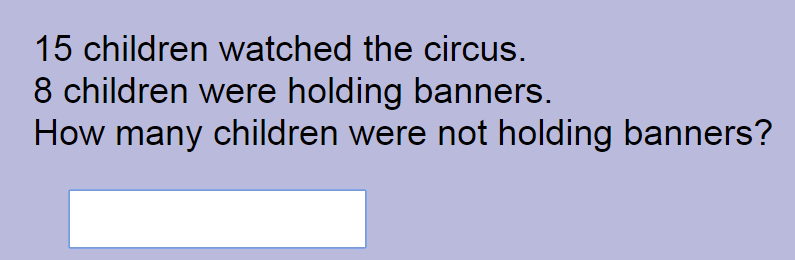### Calculations, Word Problems, and Algebra

Learning mathematics can be divided into several domains. Even in the early elementary years, with regard to numeracy, three separate domains can be defined. First, pupils need to understand magnitude as expressed in numbers. Second, pupils need to acquire skills in combining numbers, as in addition and subtraction. Third, pupils need to be able to process text and construct a number sentence to calculate the unknown. These three domains: understanding, calculation, and solving word problems are very important in early elementary mathematics.

At first glance, the domains may not seem entirely separate from each other. Having a "feel" for numbers, as observed even in higher education science classes, may help in both calculations as well as solving word problems. And understanding numbers by itself can manifest in different shades. For instance, the two questions shown below are quite different although both are simply assessing an understanding of numbers:Above copied from IXL

Calculations and word problems, however, can be more easily distinguished from each other. Calculations are just straight addition and subtraction exercises, as demonstrated in the following example:Above copied from Momonix

Word problems, on the other hand, require a student to translate text into a mathematical sentence:Above copied from Mathplayground
The above text requires a student to recognize that the text is likewise asking the question "15 - 8 = ?". Here, at first glance, it could be suggested that solving word problems is simply a wider domain and calculation is simply one of the parts. After all, solving word problems do require calculations.

However, pedagogically, calculations and word problems can be vastly different. Interventions designed to hone a student's calculation skills may include practice in addition and subtraction, recognition of number families, associative operations, and other strategies. Addressing word problems, on the other hand, begins with language comprehension. A recent study published in the Journal of Educational Psychology shows that these two are indeed separate domains and are quite independent from each other.

As the above abstract states, "...calculation intervention improve calculation outcomes but not word-problem outcomes, word-problem intervention enhanced word-problem but not calculation outcomes...." This distinction makes it clear that pupils need to be taught and assessed in both domains.

The above study likewise points out that word-problem intervention may be providing a stronger route to algebra than calculations do, but I would exercise caution with this finding as algebra also involves these two separate domains:

Calculation:

Word Problem:

Nevertheless, I strongly agree with the author's major conclusion:

"...calculation and word-problem performance at second grade appear to represent distinct aspects of mathematical cognition and indicate the need to address calculation and word-problem performance deliberately and explicitly in research and practice."